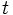Intermediate value property

(diff) ← Older revision | Latest revision (diff) | Newer revision → (diff)
A function$f$ is said to satisfy the intermediate value property if, for every$a < b$ in the domain of$f$, and every choice of real number$t$ between$f(a)$ and$f(b)$, there exists$c \in [a,b]$ that is in the domain of$f$ such that$f(c) = t$.